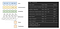# TensorFlow NLP Classification Examples

In the last article, we present TensorFlow coding examples on computer vision. Now, we will focus on NLP classification and BERT. Here is the list of examples, tested with TF 2.4.0 released in Dec 2020. These examples are originated from the TensorFlow Tutorial.

• IMDB files: Sentimental analysis with dataset mapping & Embedding,
• IMDB files: TensorBoard & Sentimental analysis with Embedding & a data preprocessing layer,
• IMDB TF Dataset: Sentimental analysis with pre-trained TF Hub Embedding,
• IMDB TF Dataset: Sentimental analysis with Bi-directional LSTM,
• Illiad files classification using data processing with tf.text & TextLineDataset,

# IMDB files: Sentimental analysis with dataset mapping & Embedding

This example performs sentimental analysis on IMDB movie reviews — classify them as pos for positive reviews and neg for negative reviews.

• transforming data using dataset pipelining mapping,
• a Sequential model composed of embedding and dense layers.

Load and prepare IMDB data files:

After removing un-wanted directories, the data directory becomes:

Prepare datasets using files from multiple directories (each directory contain samples from the same class: pos or neg):

Text preprocessing with standardization and TextVectorization (line 79–81):

In line 69, the text vectorized layer (vectorize_layer) will adapt to the corpus of the training dataset to set up the vocabulary and the word indexes. So given a standardized sentence with 10 words, it generates a sequence of 10 integers. So “what side you …” is converted to say (79, 3, 4, 23, …).

Optimize dataset:

Model creation, training & evaluation:

Here is a summary of the model.

Export a probability model:

# IMDB files: TensorBoard & Sentimental analysis with Embedding & a data preprocessing layer

In this example,

• data preprocessing is done as a layer in a model instead of using data pipeline mapping, and
• logging information into TensorBoard.

First, the boilerplate code for loading data and preparing datasets.

Next, we create the TextVectorization layer and adapt it to the training dataset.

Include the TextVectorization layer into the model. Train the model and log TensorBoard information with a callback. We can access this information later with “tensorboard -logdir logs” (where “logs” is the TensorBoard log directory in line 76).

We can save the embedding weights and vocabulary.

Metadata.tsv contains the vocabulary — one word a line and vectors.tsv contains the vector representation for each word.

We can view this embedding information with projector.tensorflow.org by uploading both files in the load button below.

# IMDB TF Dataset: Sentimental analysis with pre-trained TF Hub Embedding

In this sentimental analysis:

• data comes from the TensorFlow datasets,
• the model uses a pre-trained embedding layer from the TF hub, and
• we add dense layers to a Sequential model as a classification head.

A common practice is to wrap a pre-trained TF Hub model with hub.KerasLayer (line 20).

Here is the model:

# IMDB TF Dataset: Sentimental analysis with Bi-directional LSTM

In this example,

• data is loaded from TF datasets,
• use a TextVectorization with builtin standardization,
• include the TextVectorization (encoder) inside a model, and
• use bidirectional LSTM layers.

Here is the model summary.Left diagram source

Then, we train and evaluate the model.

# Illiad files classification using data processing with tf.text, TextLineDataset (optional)

In this example, we predict the author of Illiad translations.

The samples come from three files. Each file contains Illiad translations done by the same author. Here is the code that we create a dataset for each file. Since each file comes from the same author, we give all its samples the same label (line 33). Then, we concatentate (merge) the datasets into one and shuffle the samples. The new dataset, all_labeled_data, contains the texts and the labels.

Instead of using TextVectorization, this example goes for the more complex routes. We created a tokenized dataset from all_labeled_data using the tf_text APIs. This new dataset converts text into a sequence of words.

With TextVectorization, we can adapt it with training samples to create a vocabulary and the mappings between a word and an integer index. Here, we do it manually. First, we locate 10,000 topmost frequent word and create the mappings (vocab_table in line 96).

Finally, we create the dataset that vectorizes a text into an integer sequence (one integer for each word).

Now, we build a model, train it and evaluate it.

Export the model

Now, we create an export model including the text pre-processing that can be used for production for inferencing. This model can take raw text directly without extra code for text preprocessing. We will use a TextVectorization layer in replicating the data preprocessing. In line 142 to 148, it uses the same standardizer and tokenizer and adapts to the same vocabulary (including mapping) we created before. The rest of the code rebuilds the model and makes predictions.

# Credits and References

All the source code is originated or modified from the TensorFlow tutorial.

Deep Learning